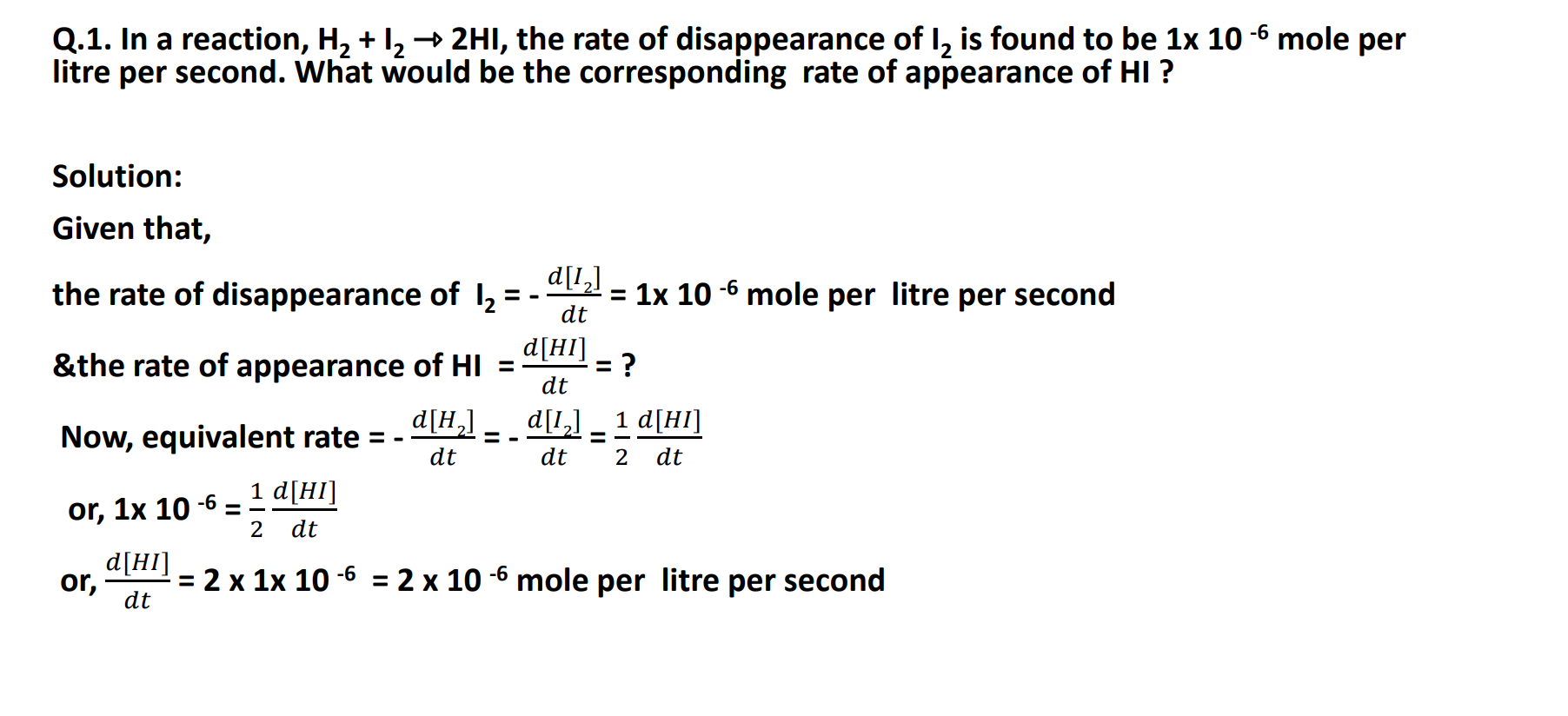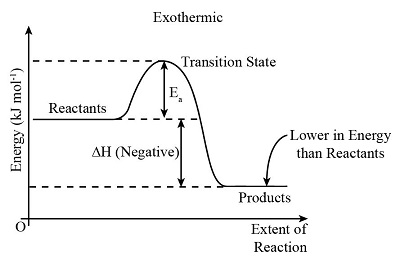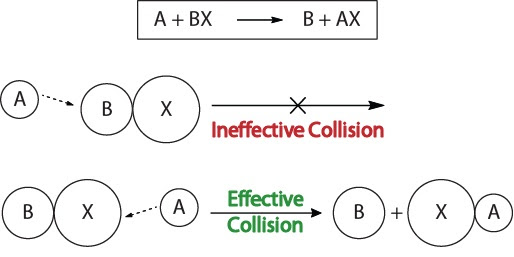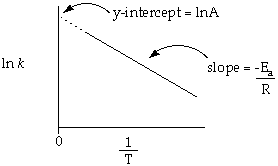Welcome to Narayannepal.com.np

# Chemical Kinetics : Class 12 Physical Chemistry Notes

Unit 3: Chemical Kinetics Class 12 Chemistry Complete Notes, Question answer, MCQs, Exercise for NEB Exams.
Chemicals Kinetics Course content
3.1 Define chemical kinetics.
3.2 Explain and use the terms rate of reaction, rate equation, and rate constant.
3.3 Explain qualitatively factors affecting the rate of reaction
3.4 Use collision theory to explain how the rate of a chemical reaction is influenced by temperature, concentration, and particle size.
2.5 Explain the meaning of the term activation energy and activated complex.
3.6 Derive and explain the integrated rate equation and half-life for zero, and first-order reactions.
3.7 Construct and use rate equations to calculate an initial rate using concentration data.
3.8 Explain the significance of the Arrhenius equation and solve the related problems.
3.9 Explain and use the term catalyst and catalysis (homogenous, heterogeneous).
3.10 Describe enzyme as the biological catalyst.
3.11 Explain the role of catalyst in the reaction mechanism.
3.12 Solve related numerical problems based on rate, rate constant, and order of zero and first-order reactions.

## Unit 3:Chemical Kinetics

The branch of physical chemistry that deals with the rate and mechanism of a chemical reaction is known as chemical kinetics.

## Rate of reaction

The Change in concentration of the reactant or product per unit time is known as the rate of reaction.

In terms of reactant, it is defined as the decrease in the concentration of reactant per unit of time. But in terms of product, it is defined as the increase in the concentration of product per unit of time.

Let us consider a general reaction where reactant “A” gives a product “B”.
A --------⇾B----(i)
let, Initially (T1),      [A1]         [B1]
finally ( T2),              [A2]         [B2]

Now,
rate of reaction = Change in concentration of A / Time Taken
(in terms of reactant)
or, Rate= [A2]-[A1] / T2-T1
or, Rate = -[A] / T -----(ii)
Then –ve sign indicates the decrease in the concentration of the reactants with the time of the reaction.
Again,
rate of reaction =Change in concentration of B / Time Taken
(in terms of products)
or, Rate= [B2]-[B1] / T2-T1
or, Rate = ∆ [B] / ∆T -----(iii)
From (ii) & (iii),
Now, the Rate of reaction = - 𝑑[𝐴]/𝑑𝑡 = 𝑑[𝐵]/𝑑𝑡
[Here, negative signs indicate that concentration decreases]

### Types of the rate of reaction

There are three types of rate of reaction, they are:

1. Initial rate: If the rate of the reaction is measured at the initial time of a reaction, then it is known as the initial rate. ( where t⇾0)
2. Average rate: It is defined as the change in concentration of reactant or product in a unit interval of time (the time interval ∆t is finite and measurable). Average rate = ∆𝑋 / ∆t
3. Instantaneous rate: The instantaneous rate of a reaction is defined as the change in concentration of the reactants or products at a given point in time i.e. it is the rate of a reaction when the change in time tends to zero. Instantaneous Rate= 𝑑𝑥 / 𝑑𝑡.
The instantaneous rate of time is calculated as the slope of the tangent drawn from the corresponding point curve of the reactant or product.
Instantaneous rate of a time at t = slope of Tan.

### Unit of the rate of reaction

we know that, the rate of reaction= change in concentration/change in time
But, we know that unit of concentration is molL-1, and the unit of time is second minutes or hours. so, the unit of rate is mol L-1/time.

=     Mol L-1 / Time
=     Mol L-1 Time-1
i.e. Mol L-1 S -1 or Mol L-1 min-1 or Mol L-1 Hr-1
(unit of time may be different for various reactions)

### Factors affecting the rate of reaction

The following factors influence the rate of reaction:

1. Concentration of reactant: The rate of reaction with the increase in the concentration of reactant except for zero-order reaction. The change of collision between reactant molecules to give products increases with the increase in the number of reactant particles.

Rate = 𝑐𝑜𝑛𝑐𝑒𝑛𝑡𝑟𝑎𝑡𝑖𝑜𝑛 / 𝑡𝑖𝑚e

which indicates that the rate of reaction is directly proportional to the concentration of reactant.

2. Temperature: The increase in temperature generally increases the rate of reaction because increasing temperature increases the Kinetic energy of reactant molecules and more fraction of molecules becomes able to cross-activation energy barrier to give the product.

Experimentally, It has been found that the rate of reaction increases by 2 to 3 times with every rise in temperature by 10' C.

3. Surface area of reactant: The rate of reaction increases with an increase in the total surface area since the reactive site increases. For example, the reaction between a marble piece ( CaCO3 ) and dil. HCl is very slow but CaCO3 powder with dil. HCl is very fast.4. Catalyst: The rate of a reaction can be increased by adding a catalyst. A catalyst is a substance that provides a new path for the reaction with lower activation energy and speeds up the reaction.

A positive catalyst increase the rate of reaction but a negative catalyst decreases the rate of reaction.

5. Nature of reactant: Reactions having ionic reactants in an aqueous solution have a high rate of reaction, whereas reactions having covalent reactants have a low rate of reaction. In an aqueous medium (or solution) ionic compounds are completely ionized before their reaction, so are mostly instantaneous.

Moreover, reactions in the gaseous or liquid phase have a high rate of reactions than the solid phase. example: Rusting of Iron is a slow process (low rate of reaction) because it involves the reaction between iron (Fe) and moist air (O2 + H2O).

6. Light: The rate of photochemical reactions is directly proportional to the intensity of the light, i.e. the amount of product of the photochemical reaction is more at a high intensity of sunlight.

Example: 𝐻2 + 𝐶𝑙2-----------> 2HCl

Very slow rate of reaction in absence of sunlight but rapidly takes place in presence of sunlight.

## Rate of Reaction in terms of  Stoichiometry

The rate of reaction can be expressed in terms of the rate of formation of product, or rate of disappearance of reactant.
Let us consider the reaction,
H2 + I2 -----> 2HI

Rate of formation of HI = 𝑑[HI] /𝑑t

Rate of disappearance of H2 = - 𝑑[H2] /𝑑 t

Rate of disappearance of I2 = - 𝑑[I2] / 𝑑t

Equivalent rate Expression:

- 𝑑[H2] /𝑑 t = - 𝑑[I2] / 𝑑t =1/2(𝑑[HI] /𝑑t )

The number of moles of reactant and product isn't the same.

similarly, the rate of disappearance of reactant and the rate of formation is also not the same.

To write the equivalent rate expression, the rate of disappearance and formation are divided by their stoichiometric coefficient in a balanced chemical equation.

2A + 3B ⇾ 4C

Here, the rate of disappearance of A= - 𝑑[𝐴] / 𝑑𝑡

the rate of disappearance of B = - 𝑑[𝐵] / 𝑑𝑡

& the rate of appearance of C = 𝑑[𝐶] / 𝑑𝑡

Now, equivalent rate = - 1 /2 (𝑑[𝐴] / 𝑑𝑡) = - 1 /3(𝑑[𝐵] / 𝑑𝑡) = 1/ 4(𝑑[𝐶] / 𝑑𝑡)## Rate equation or Rate law

An equation that expresses the relation between the rate of the reaction and the concentration of the reactant present in the given chemical reaction is called the rate equation or rate law.

Let us consider a general reaction

nA ⇾ product

The rate equation of this reaction

R α [A]^n
R= k[A]^n

Where k=proportionality constant known as the rate constant
& n= power of concentration of A.

### Order or reaction

The sum of the power of concentration in the rate law equation is known as the order of the reaction.

Let us consider A and B reactant

Now, A + B --> product
Rate = K [A]^x. [B]^y
Order of reactant = x + y

The order can also be represented independently as 'x' order to A and 'y' order to B.
The values of x & y can be experimentally determined only.

#### Types of reaction on the basis of Order

If,
• value of (m + n) = 0, the reaction is zero order
• value of (m + n) = 1, the reaction is first order
• value of (m + n) = 2, the reaction is second order
•  value of (m + n) = 3, the reaction is third order

### Molecularity of a Reaction

Molecularity of a reaction depends upon the reactant involved in the chemical reaction so, Molecularity of a reaction is the total number of species ( atoms, ions, and molecules) combining simultaneously to give the product.

It is obtained by summing the stoichiometric coefficients of a balanced chemical reaction.

Let us take a general reaction as follows:
a.A +b.B ----------------> C

Now, the sum of a and b i.e. value of (a + b) is the molecularity of the above reaction.

#### Types of reaction on the basis of molecularity

There are three types of reaction on the basis of molecularity :

1. Unimolecular Reaction  (Molecularity=1)
2. Bimolecular Reaction    (Molecularity=2)
3. Trimolecular Reaction   (Molecularity=3)

##### 1.Unimolecular Reaction (Molecularity = 1)

Value of (a + b) = 1, the reaction is unimolecular reaction.

N2O5 ----------> 2 NO2 + 1 /2 O2

##### 2.Bimolecular Reaction    (Molecularity=2)

Value of (a + b) = 2, the reaction is bimolecular reaction.

H2 + I2 ----------> 2 HI

##### 3.Trimolecular Reaction   (Molecularity=3)

Value of (a + b) = 3, the reaction is is trimolecular reaction.

2NO + O2 ----------> 2 NO2

### Differences between Molecularity and Order of reaction

Molecularity  Order of reaction
It is the sum of the number of molecules present in the rate-determining step of the balanced chemical equation.  It is the sum of the power of concentration term on which the rate of reaction actually depends.
It is determined theoretically. It is an experimentally determined value.
Its value can never be fractional & zero. It is always in simple whole numbers. It can be a fractional, zero, or whole number.
It is invariant (can't be changed with the external conditions) It can be changed with an external condition
It has no unit. It has a certain unit.

# Not all reactions lead to the formation of products.

### Two Types of reaction

#### 1.Elementry ( simple ) reaction

The reaction which is completed in a single step is called a simple reaction.

The number of reacting molecules involved in the elementary reaction is called molecularity.

A  ---> product
Br2---(hv)--> 2Br * uni molecular
A + B ---> product
H2 + I2---> 2HI * bi molecular

#### 2. Complex reaction

A reaction that completes in more than one step is the complex reaction.

The number of reacting molecules involved in the rate is determined in the slowest step.

step1: 2N2O5 ----> 2NO2 + 2NO3 (Slow)
step2: NO2 + NO3 ---> NO + NO2 + O2 (slowest)
step3: NO + NO3----> 2NO2(fast)

>>>>2N2O5------> 4NO2 + O2

## Rate Constant

The rate constant is defined as the proportionality constant which explains the relationship between the molar concentration of the reactants and the rate of a chemical reactant.

The rate constant is denoted by K and is also known as the reaction rate constant or reaction rate coefficient. It is dependent on the temperature.

There are two possible ways to calculate the rate constant and they are

1. Using the Arrhenius Equation.
2. Using the molar concentration of the reaction and the order of the reaction.

### Some Important Characteristics of the rate constant are given below:

i. Rate constant is the measure of the rate of reaction action, Larger the value of the rate constant, the greater will be the rate of reaction.

ii. Rate constant is independent of the concentration of reactants.

iii. Rate constant is the characteristic value of each reaction. Different reactions have different values of rate constants at a particular temperature.

iv. Rate constant depends upon the temperature Changing the temperature, the value of the rate constant is also changed.

v. The unit of rate constant depends upon the order of the reaction.

K= Rate/[A]^x.[B]^y

when A=1=B then,

K = rate ,

The rate constant is the rate reaction condition when the reactant is unity.

### Unit of Rate Constant

Rate = mol L-1 Sec-1

Let us consider a simple reaction:

A------> product

The rate law expression is

Rate = K [A]^x

1. For zero order, x=o then

K.1=rate

K= mol L-1 Sec-1

2. For the first order reaction, x=1 then

K = rate/ A

K=mol L-1 Sec-1 / mol L-1

K= sec-1

so on.

Note: you can add 1 to the power of L and that number will be the order of the reaction.

## Rate Equation (Integrated rate law)

### Zero Order Reaction

Reactions in which the concentrations of the reactant do not affect the rate of reaction or the reaction in which concentrations of the reactant do not change with time are called zero-order reactions. The reaction between hydrogen & chlorine in absence of light.

H2 + Cl2 ⇾ 2HCl

#### Half-life for Zero Order Reaction

A time at which half of the concentration of reactant is changed into the product or the time at which half of the reaction is completed is called the half-life of the reaction. It is denoted by a symbol t1/2 or t0.5 Half-life of zero order reaction We have an integrated rate equation of zero order reaction.

### First order reaction

The reaction in which the rate of reaction is directly proportional to the concentration of only one reactant is called a first-order reaction. For example, Hydrolysis of the ester of the acidic medium.

CH3COOC2H5 + H2O--->CH3COOH + C2H5OH

This reaction is also an example of a pseudo-first-order reaction.

### Activation Energy (Ea) and Activated Complex

The minimum amount of Energy required by the reactant to change into the product is called activation energy

or,

The minimum amount of energy that must be supplied to the reactants to enable them to cross over the energy barrier is called activation energy.Fig: energy profile diagram for the exothermic reaction

If the activation energy is higher in a reaction then slower will be the rate of reaction.

The highest energy state in a chemical reaction in which old bonds are broken and new bonds are formed is called Transition State.

A reaction having Lower activation energy occurs fast because a large number of molecules will soon cross the energy barrier. similarly, the reaction with higher activation energy will be slow.

The reaction having activation energy Zero is nearly Explosive.

## collision theory of reaction rate

According to collision theory, the number of collisions between reactant molecules determines how quickly a chemical reaction proceeds.

To occur a reaction there must be a collision between the reacting molecules But all the molecules which collide do not necessarily give products.

Only a certain fraction of the total number of collisions are capable to give products, such collisions are called effective collisions.

### condition for effective collisions are:

1. The reacting species should have sufficient kinetic energy to break the chemical bond in the reacting molecules and overcome the energy barrier
2. The colliding species should have proper orientation so that the old bonds existing between reacting species break and new bonds are formed.1. Ineffective collision 2. Effective collision due to proper orientation.

Thus, the collision in which the colliding molecules don't possess the minimum energy for effective collision (Threshold energy) or proper orientations doesn't form the product.

Threshold Energy: The minimum amount of energy required for reacting molecules to convert into the product is called threshold energy.

Threshold Energy = Activation Energy + Average Energy

Et= Ea + Eavg .

## Arrhenius Equation

The effect of temperature on the rate constant of reaction was studied by Arrhenius and the equation is expressed.

k = Ae-Ea/RT    -----(i)

Where K = rate constant

A= Constant, Called frequency factor (Arrhenius frequency factor)

Ea = Activation energy

R = Universal gas constant (8.314 J / mol K)

T= absolute temperature

Validity of Arrhenius equation

we have,

k = Ae-Ea/RT

Taking Ln Common on both sides we get,

Ln(k) =Ln ( A e-Ea/RT

or, Ln k = Ln A + Ln e-Ea/RT

or, Ln k = Ln A - Ea / RT -----(ii)

Eqn. (ii) is in the form of y = mx + c where, y=Ln k , C= Ln A , m=Ea/R , x=1/T so , when we plot the graph between Ln k v/s 1/T we get a straight line with slope -Ea/R & intercept Ln A.

From the intercept we can determine the values of A & from the slope, we can determine the values of activation energy.Ln k & 1/T graph

If the reaction is carried out at two different temperatures T1 & T2. then Eqn (ii) can be modified as :

or, Ln k1 = Ln A - Ea / RT1   -----(iii)
or, Ln k2 = Ln A - Ea / RT2   -----(iv)

Now, subtracting Eqn. (iii) from Eqn. (iv) we get

Ln K2 - Ln K1 =Ln A - Ea / RT2 - Ln A +Ea / RT1

or, Ln K2/K1 = Ea/ R [ 1/ T1 - 1/T2 ]

or, 2.303 logK2/K1 = Ea/ R [ 1/ T1 - 1/T2 ]

or, 2.303 logK2/K1 = Ea/ R [ (T2 - T1) / T1. T2 ]

## catalyst and catalysis

### Catalyst

The chemical substance which alters the RoR remains unchanged at the end of the Reaction, the substance is the Catalyst. Examples,

1. Nickel is used to creating margarine by hydrogenating palm oil. Iron, or
2. Fe is used in the Haber process. (the production of ammonia)

### catalysis

Catalyst is the chemical substance that alters the RoR and remains unchanged at the end of the Reaction, this process is called catalysis.

Depending upon the phase of the catalyst and Reactants. Catalyst is classified into two types:

#### i. Homogeneous Catalyst:

If the phase catalyst and reactant are in the same phase it is then called a Homogenous catalyst.

2 SO2 (g) + O2 ----NO(g)--> 2SO3

#### ii. Heterogeneous Catalyst:

If the phase of catalyst and reactant are in different phases then it is called Heterogenous Catalyst.

N2 + 3 H2 (gas) --Fe(solid)--> 2NH3

[ Phase ≠State]
water and oil are also in different phases.
phase = mechanically separated

### Enzyme catalyst

Enzymes are complex nitrogenous organic compounds that act as a catalyst in a living organism. They are protein that acts as biological catalyst. ( biocatalyst)Reaction with or without Enzymes catalyst

### Why is an enzyme a biological catalyst?

Because they speed up biochemical reactions within an organism, enzymes are also referred to as biocatalysts. Enzymes are referred to as biocatalysts because they speed up chemical reactions without altering their equilibrium state.

Example:

#### 1. Hydrolysis of sucrose is catalysis by invertase

C12H22O11 + H2O --Invertase-->  C6H12O6 (Glucose)C6H12O6 (Fructose)

Zymase: An Enzyme produced by living yeast

#### 2. Convert Glucose into ethyl alcohol.

C6H12O6--Zymase--> C2H5OH + H2O

### Types of Catalyst

#### 1. Positive Catalyst:

A positive catalyst is the one that increases RoR by providing the path which has lower activation Energy.

2KClO3--MnO2--> 2KCl + 2O2

MnO2 is the positive catalyst.

#### 2. Negative catalyst:

A negative catalyst is one that decreases RoR by increasing activation Energy.

2H2O2  --glycerol--> 2H2O + O2

Glycerol acts as a negative catalyst.

Getting Info...